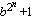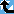# Generalized Fermat Divisors (base=5)

The Prime Pages keeps a list of the 5000 largest known primes, plus a few each of certain selected archivable forms and classes. These forms are defined in this collection's home page.

The numbers Fb,n =(with b an integer greater than one) are called the generalized Fermat numbers. (In the Prime database they are denoted GF(b,n) to avoid the use of subscripts.) It is reasonable to conjecture that for each base b, there are only finitely many such primes.

As in the case of the Fermat numbers, many have interested in the form and distribution of the divisors of these numbers. When b is even, each of their divisors must have the form

k.2m+1
with k odd and m>n. For this reason, when we find a large prime of the form k.2n+1 (with k small), we usually check to see if it divides a Fermat number. For example, Gallot's Win95 program Proth.exe has this test built in for a few choices of b.

The number k.2n+1 (k odd) will divide some generalized Fermat number for roughly 1/k of the bases b.

###Record Primes of this Type

rankprime digitswhowhencomment
13 · 216408818 + 1 4939547 L5171 Oct 2020 Divides GF(16408814, 3), GF(16408817, 5)
29 · 211158963 + 1 3359184 L4965 Mar 2020 Divides GF(11158962, 5)
33 · 210829346 + 1 3259959 L3770 Jan 2014 Divides GF(10829343, 3), GF(10829345, 5)
439 · 26164630 + 1 1855741 L4087 Aug 2020 Divides GF(6164629, 5)
521 · 26048861 + 1 1820890 L5106 Jun 2020 Divides GF(6048860, 5)
63 · 25082306 + 1 1529928 L780 Apr 2009 Divides GF(5082303, 3), GF(5082305, 5)
715 · 24800315 + 1 1445040 L1754 Oct 2019 Divides GF(4800313, 3), GF(4800310, 5)
8163 · 23874556 + 1 1166360 L5646 Jan 2023 Divides GF(3874552, 5)
935 · 23587843 + 1 1080050 L1979 Jul 2014 Divides GF(3587841, 5)
103 · 22291610 + 1 689844 L753 Aug 2008 Divides GF(2291607, 3), GF(2291609, 5)
117 · 22167800 + 1 652574 g279 Apr 2007 Divides Fermat F(2167797), GF(2167799, 5), GF(2167799, 10)
123 · 22145353 + 1 645817 g245 Feb 2003 Divides Fermat F(2145351), GF(2145351, 3), GF(2145352, 5), GF(2145348, 6), GF(2145352, 10), GF(2145351, 12)
1325 · 22141884 + 1 644773 L1741 Sep 2011 Divides Fermat F(2141872), GF(2141871, 5), GF(2141872, 10); generalized Fermat
1471 · 21873569 + 1 564003 L1223 Sep 2011 Divides GF(1873568, 5)
153 · 21832496 + 1 551637 p189 Jul 2007 Divides GF(1832490, 3), GF(1832494, 5)
1645 · 21779971 + 1 535827 L1223 Aug 2011 Divides GF(1779969, 5)
175 · 21777515 + 1 535087 p148 Apr 2005 Divides GF(1777511, 5), GF(1777514, 6)
183803 · 21553013 + 1 467508 L1957 Jan 2020 Divides GF(1553012, 5)
195529 · 21430926 + 1 430756 L3035 Jan 2017 Divides GF(1430925, 5)
2015 · 21418605 + 1 427044 g279 Apr 2006 Divides GF(1418600, 5), GF(1418601, 6)

###References

BR98
A. Björn and H. Riesel, "Factors of generalized Fermat numbers," Math. Comp., 67 (1998) 441--446.  MR 98e:11008 (Abstract available)
DK95
H. Dubner and W. Keller, "Factors of generalized Fermat numbers," Math. Comp., 64 (1995) 397--405.  MR 95c:11010
RB94
H. Riesel and A. Börn, Generalized Fermat numbers.  In "Mathematics of Computation 1943-1993: A Half-Century of Computational Mathematics," W. Gautschi editor, Proc. Symp. Appl. Math. Vol, 48, Amer. Math. Soc., Providence, RI, 1994.  pp. 583-587, MR 95j:11006
Riesel69
H. Riesel, "Some factors of the numbers Gn = 62n + 1 and Hn = 102n + 1," Math. Comp., 23:106 (1969) 413--415.  MR 39:6813